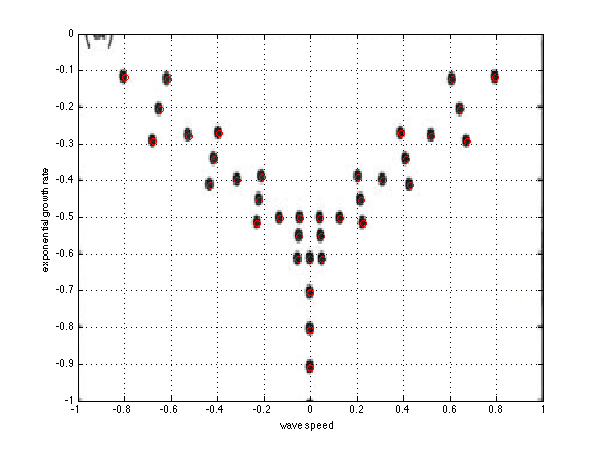# Stability of Couette flow in primitive variables

This code is very much like poiseuille_uvp.m except that we now have as well the velocity w in the spanwise direction. There is thus one more variable. The system is Fourier transformed in both the x and the z direction which are homogeneous directions, such that differentiation in these directions are changed to i \alphaI and i\betaI, where \beta is the wavenumber in the spanwise direction. We validate the computation against a figure in the litterature for \alpha=1 and \beta=1, an oblique wave.

Here is the sketch of the flow configuration:plane Couette flow

clear all; clf;

alpha=1;    % the wave number in x
beta=1;    % the wave number in z
L=2;        % the height of the domain, from -1 to 1
Re=1000;    % the Reynolds number
n=100;      % the number of grid points

% differentiation and integration
scale=-2/L;
[y,DM] = chebdif(n,2);
D=DM(:,:,1)*scale;
DD=DM(:,:,2)*scale^2;
y=y/scale;
Z=zeros(n,n); I=eye(n);
INT=([diff(y)',0]+[0,diff(y)'])/2;

% renaming the differentiation matrices
I=eye(n); Z=zeros(n,n);
dy=D; dyy=DD;
dx=i*alpha*I; dxx=-alpha^2*I;
dz=i*beta*I; dzz=-beta^2*I;
Delta=dxx+dyy+dzz;

% base flow
U=y; Uy=1+0*y;
S=-diag(U)*dx+Delta/Re;

% the matrices
A=[ ...
S,  -diag(Uy),  Z,  -dx; ...
Z,  S,  Z,  -dy; ...
Z,  Z,  S,  -dz; ...
dx, dy, dz, Z];
E=blkdiag(I,I,I,Z);

% locations on the grid
u=1:n; v=u+n; w=v+n; p=w+n;

% boundary conditions
III=eye(4*n);
DDD=blkdiag(dy,dy,dy,dy);

loc=[u(1) u(n) v(1) v(n) w(1) w(n) ];
C=III(loc,:);
E(loc,:)=0;  A(loc,:)=C;


# Validation of the results

We plot our eigenvalues on the figure from Schmid & Henningson’s book “Stability and transition in shear flows”, page 67, figure 3.3.

% loading the scanned figure for comparison
x=linspace(-1,1,s(2));
y=linspace(0,-2,s(1));
image(x,y,a); axis xy
hold on

% computing eigenmodes and plotting
[U,S]=eig(A,E);
s=diag(S);  [t,o]=sort(-real(s)); s=s(o); U=U(:,o);
rem=abs(s)>1000; s(rem)=[]; U(:,rem)=[];
plot(-imag(s)/alpha,real(s),'ro')
grid on
axis([-1,1,-1,0]);
xlabel('wave speed'); ylabel('exponential growth rate')The spectra of the Couette flow at Reynolds 1000

%}